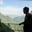Related Tags

matrix
r programming
data structures
communitycreator

# What is a matrix data structure in R?AKASH BAJWA

A matrix is an R object which is similar to a vector, arranged in a two-dimensional rectangular representation. It has some additional attributes with it. All these attributes can be accessed by using the attribute() method i.e., dimensions. The dim() method returns the dimensionality (rows & columns) of the argument value, i.e., matrix.

Matrices can be of any type, however we mostly deal with numerical data for different computations (data analysis). Primarily, we use matrices with numerical values in R, as it is a language specialized for data analysis.

### Syntax

matrix(data, nrow, ncol, byrow, dimnames)


### Parameters

• data: is the input vector that are data attributes in the matrix.
• nrow: number of rows in the matrix.
• ncol: number of columns in the matrix.
• byrow: is a logical type. If it is TRUE, then the input attributes are arranged by row.
• dimname: is the names that are assigned to the rows and columns.

### Demo Code

This code snippet below is generating two matrices, M and N (line 2 and 9). Each matrix has four rows nrow = 4 and data values from 3 to 14 c(3:14). It will generate a 4x3 matrix, because we have row = 4 and there is a total of 12 values between 3 and 14.

In order to set row and column names, you can use the code below on lines 9 and 10. These lines will help to change the names of rows and columns.

# Elements are arranged by row
M <- matrix(c(3:14), nrow = 4, byrow = TRUE)
# c(3:14) meas we have values in matrix
# 3,4,5,6,7,8,9,10,11,12,13,14
cat("Matrix M",sep =" \n ")
print(M)

# Give the column and row names
rownames = c ("row1", "row2", "row3", "row4")
colnames = c ("col1", "col2", "col3")

# c(3:14) meas we have values in matrix
# 3,4,5,6,7,8,9,10,11,12,13,14
N <- matrix (c (3:14), nrow = 4, byrow = TRUE, dimnames = list (rownames, colnames))
cat("Matrix P",sep =" \n ")
print(N)

### 1. Accessing Elements of a Matrix

To access elements of a matrix, we can use indexing [,]. We can mention row number and column number to access them randomly.

### Demo Code

• Case#1: Passing only Row number i.e [2,]

It results in the 2nd row.

• Case#2: Passing only Column number i.e [,3]

It results in the 3rd column.

• Case#3: Passing both values i.e [1,2]

It results in the element at row= 1 and col= 2 index.

• Case#4: Empty index values i.e [,]

This will print the whole matrix.

# Give the column and row names
rownames = c ("row1", "row2", "row3", "row4")
colnames = c ("col1", "col2", "col3")
# Create the matrix
P <- matrix (c (3:14), nrow = 4, byrow = TRUE, dimnames = list (rownames, colnames))
# Accessing the elements at 2nd Row
cat("Case#1:",sep =" \n ")
print (P [2,])
# Accessing the elements at 3rd column
cat("Case#2:",sep =" \n ")
print (P [,3])
# Accessing  the element at 1st row and 2nd col.
cat("Case#3:",sep =" \n ")
print (P [1,2])
# Access whole matrix.
cat("Case#4:",sep =" \n ")
print (P [,])


### 2. Matrix Computations

Multiple mathematical operations can be performed on the matrices and the result is also a matrix. The dimensions (rows by columns) should be exactly identical for the matrices that are used in the operation.

This code demonstrates the addition of matrices using R as the programming language. The same rule of linear algebra will apply. Two matrices are confirmable for addition or subtraction when they have same order. matrix_A and matrix_B have the same dimensions So, on lines 9 and 13, they are being added and subtracted respectively.

# Create two 2 by 3 matrices
matrix_A <- matrix (c (3, 9, -1, 4, 2, 6), nrow = 2)
cat ("Matrix-A","\n")
print(matrix_A)
matrix_B <- matrix (c (5, 2, 0, 9, 3, 4), nrow = 2)
cat ("Matrix-B","\n")
print(matrix_B)
out <- matrix_A + matrix_B
print(out)
# Difference the matrices
out <- matrix_A - matrix_B
cat ("Matrices Subtraction is: ","\n")
print(out)


#### Matrix Multiplication & Division

On highlighted lines, index-by-index multiplication and division is occurring between Matrix-A and Matrix-B. During the division process, divide by zero case can occur. So, such index will be replaced by -Inf.

# Create two 2 by 3 matrices
matrix_A <- matrix (c (3, 9, -1, 4, 2, 6), nrow = 2)
cat ("Matrix-A","\n")
print(matrix_A)
matrix_B <- matrix (c (5, 2, 0, 9, 3, 4), nrow = 2)
cat ("Matrix-B","\n")
print(matrix_B)
# Multiply the matrices
out <- matrix_A * matrix_B
cat ("Multiplication:","\n")
print(out)
# Divide the matrices
out <- matrix_A / matrix_B
cat ("Division:","\n")
print(out)


RELATED TAGS

matrix
r programming
data structures
communitycreator

CONTRIBUTORAKASH BAJWA
RELATED COURSES

View all Courses

Keep Exploring

Learn in-demand tech skills in half the time